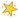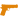BOT#97 - CS.MONEYCSGO Trade Bot{LINK REMOVED}
Trade Link
Currently Offline
Warning, read this message please!
This account used only on the CS.MONEY trading website
If any other website refers to this account - is a fraud

All sites with deposit/withdraw systems - is a fraud

Be careful with your skins :)
Items Up For Trade
1,293
Items Owned
51,190
Trades Made
3
Market Transactions
Favorite Group
CS.MONEY - Public Group
CS.MONEY
209,847
Members
2,807
In-Game
17,189
Online
15,170
In Chat
Comments
La re vivis pollito Apr 8 @ 8:08am
ESTAFA!!!!!!
-dieMONDZ Nov 21, 2020 @ 3:17am
HEY BOT! Give me please a knife))))))))))))))))))))))))))))))))))))))))))))))))))))))))))))))))))))))))))))))))))))))))))))))))))))))))))))))))))))))))))))))))))))))))))))))))))))))))))))))))))))))))))))))))))))))))))))))))))))))))))))))))))))))))))))))))))))))))))))))))))))))))))))))))))
nopo Trade Banned Sep 16, 2020 @ 11:28am
Hello I'm interested in a deal with you, add me please I can't since I've reached my weekly invite limit. TySamit May 28, 2020 @ 1:23am
Important!
Your Steam id 64 won in random selection.
Please go to the website https://onegg.fun
Be sure to use the code "ZW66OW6" by which we check the winner.
After identifying the code, you will instantly gain access to winning items.
You can choose one of several items:
* Falchion Knife | Boreal Forest
* Ursus Knife | Stained
* Huntsman Knife | Doppler
* StatTrak™ Gut Knife | Rust Coat
* Bayonet | Doppler
* Navaja Knife | Blue Steel
* Talon Knife | Doppler
* Classic Knife | Forest DDPAT
* Survival Knife | Crimson Web
* Karambit | Doppler
* Falchion Knife | Scorched
* Ursus Knife | Stained
* Karambit | Tiger Tooth
* Gut Knife | Freehand
- Flashz0r Feb 6, 2020 @ 6:56am
-rep
♕ ᴊᴏᴋᴇʀ tradeit.gg Jan 25, 2020 @ 4:01am
+rep